##### Actions

(diff) ← Older revision | Latest revision (diff) | Newer revision → (diff)

A method for isolating the algebraic part in indefinite integrals of rational functions. Let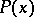and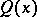be polynomials with real coefficients, let the degree ofbe less than the degree of, so thatis a proper fraction, let(1)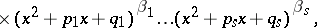where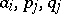are real numbers,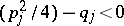,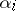andare natural numbers,,, and let(2)

Then real polynomials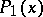andexist, the degrees of which are respectively less than the degrees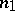andof the polynomials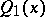and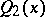, such that(3)

It is important that the polynomialsandcan be found without knowing the decomposition (1) of the polynomialinto irreducible factors: The polynomialis the greatest common divisor of the polynomialand its derivativeand can be obtained using the Euclidean algorithm, while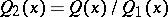. The coefficients of the polynomialsandcan be calculated using the method of indefinite coefficients (cf. Undetermined coefficients, method of). The Ostrogradski method reduces the problem of the integration of a real rational fraction to the integration of a rational fraction whose denominator has only simple roots; the integral of such a fraction is expressed through transcendental functions: logarithms and arctangents. Consequently, the rational fractionin formula (3) is the algebraic part of the indefinite integral.

The method was first published in 1845 by M.V. Ostrogradski (see ).

How to Cite This Entry: